# Astronomy 12 - Spring 1999 (S.T. Myers)

## Solutions to Problem Set #7

Solutions:
1. We use the usual relation

log( L / Lsun ) = ( 4.83 - Mv ) / 2.5

so

4.83 - Mv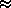2.5 [ 1.15 log( P / 1 day ) + 2.47 ]

or

Mv = -2.875 log( P / 1 day ) - 1.34

for the absolute visual magnitude of a Cepheid. The distance modulus

mv - Mv = 5 log( d / 1 pc ) - 5 + Av       ( no dust Av=0 )

gives

log( d / 1 pc ) = 0.2 [ mv + 2.875 log( P / 1 day ) + 6.34 ] = 0.2 mv + 0.575 log( P / 1 day ) + 1.27

thus for Polaris ( P = 13 days, mv = 2.3 )

log( d / 1 pc ) = 0.2 · 2.3 + 0.575 · log 13 + 1.27 = 2.37

or d = 235 pc. Note that the absolute magnitude of Polaris is

Mv = -2.875 log 13 - 1.34 = -4.54

with a luminosity of 5611 Lsun!

2. The mean density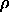= ( 3 M ) / ( 4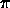R3 )

and the period-density relation

P = [ 3/ (2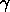G) ]1/2

give on substitution

P = [ ( 22 R3 ) / (G M ) ]1/2

or numerically

P = 6117 s · ( M / Msun )-1/2 ( R / Rsun )3/2 ( 3/ 4 )-1/2

which fills in our table:

M / Msun   R / Rsun    Mv    P (days)
5  36.3   -3.1   6.925
10  195.0   -5.9  60.96

The quantities of interest are:

log P Mv
0.840 -3.1
1.785 -5.1

and thus we solve the system of equations

-3.1 = 0.840 · a   +   b
-5.9 = 1.785 · a   +   b

which gives by subtraction

2.8 = - 0.945 · a   ==>   a = -2.963

and then

b = -3.1 - 0.840 · a   ==>   b = -0.611

by substitution. Our period-magnitude relation is thus

Mv = -2.963 log( P / 1 day ) - 0.61

(which is similar to that used in the previous problem), and

P = 20 days   ==>   log P = 1.301   ==>   Mv = -4.465

and thus the distance modulus

mv - Mv = 10.4 - (-4.465) = 14.865

gives us

log( d / 1 pc ) = 0.2 [ mv - Mv + 5 ] = 3.973   ==>   d = 9397 pc

and the star is almost 10 kpc away.

3. To locate Mira on the H-R diagram, we need its luminosity (in Lsun) and its effective temperature. For an absolute magnitude Mbol = -5 and the Sun's bolometric magnitude Mbol = +4.75 we get

log( L / Lsun ) = ( 4.75 + 5 )/2.5 = 3.9   ==>   L = 7943 Lsun

Looking at the H-R diagram on p.321 of ZG4 for example, the point

log( L / Lsun ) = 3.9         log( T ) = 3.36

is to the right of the asymptotic giant branch (AGB). Mira must be an evolved star (see also the diagram on p.353 of ZG4).

For the average temperature T = 2300 K, the average radius is

R / Rsun = ( L / Lsun)1/2 ( T / 5770 K )-2 = 439

which is in line with it being a late M supergiant. The luminosity ratio is given by the magnitude difference

Lmax / Lmin = 10 5.1/2.5 = 109.6

and so

Rmax / Rmin = ( Lmax / Lmin )1/2 ( Tmax / Tmin )-2 = 6.2

and the radius changes by a factor of 6 during the pulsation cycle!

4. For stars of the same luminosity L, they will appear to have a flux

f = L / (4d2)

and thus counting the number brighter than some limiting flux f is equivalent to counting the number closer than a limiting distance

d = [ L / (4f) ]1/2.

If the stars are distributed uniformly with density n per unit volume, then

N( >f ) = n V( d3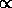( f-1/2 )3f-3/2.

Note that this is true only for a uniform volume density of sources, but is true for any luminosity function of stars.

5. The circular velocity is given by

vcirc2 = G M / R   ==>   Mv2 R

and since the velocity v is constant

M( < R )R

is the mass law. For a spherical mass "halo", the mass grows as

dM/dr = 4R2==>R-2 dM/drR-2

which is a common profile for the outer parts of massive galaxies and clusters of galaxies. Note you could have also obtained this scaling byM / R3R / R3R-2

as before.

smyers@nrao.edu   Steven T. Myers